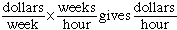Name: Tanya Who is asking: Student Level: Middle Question: I have 2 questions covert 628 kilometers to miles.(round off your answer to two decimal places.) a new clerk in your office is to earn \$200 per week. If she works 30.5 hours each week, what is her hourly rate? (round off your answer to the nearest hundreth, that is, to the nearest cent.) Hi Tanya, If I need to convert between inches/feet/miles/etc. to metric distance units I use the only conversion factor I remember, 2.54 cm = 1 inch. I also remember that there are 12 inches in a foot and 5280 feet in a mile. Thus 1 mile = 5280 feet = 5280 x 12 inches = 5280 x 12 x 2.54 centimeters The "cent" in centimeter tells me there are 100 centimeters in a meter and the "kilo" in kilometers tells me there are 1000 meters in a kilometer. Hence 1 mile = 5280 x 12 x 2.54 centimeters = (5280 x 12 x 2.54)/100 meters = (5280 x 12 x 2.54)/(100 x 1000) kilometers = 1.609344 kilometers or 1 kilometer = 1/1.609344 miles = 0.6213711 miles Thus 628 kilometers = 628 x 0.6213711 miles As in problem 1 it is the units that direct what you should do. You have 200 dollars/week and 30.5 hours/week and you want dollars/hour.and hence 200 dollars/week x (1/30.5) weeks/hour = 6.56 dollars/hour Cheers, Penny Go to Math Central